|

# 还在担心瓷砖剥落?雨虹C200瓷砖胶评测

家装瓷砖铺贴，最怕遇到的问题是什么？如果施工材料选择不当、或者施工操作不规范，那就极易造成瓷砖空鼓，使用时间一长，随着黏着力的下降，就很容易出现瓷砖剥落掉砖，如果是室内墙面还好，如果是室外墙面，高空掉砖造成人员伤亡也不是耸人听闻。无论如何，如果能够避免二次施工，相信无论是施工方、业主、物业都会更加省心。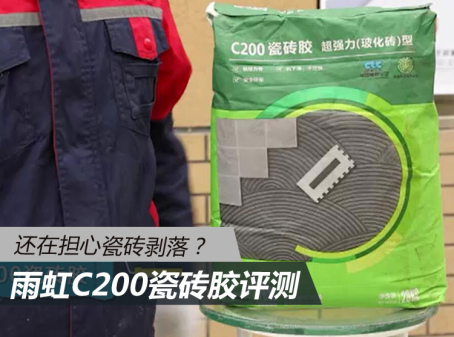撇开瓷砖铺贴施工操作规范性不说，我们在选择施工材料的时候，如果更加慎重一点，就可以防患于未然。今天我们将要评测的是在瓷砖铺贴领域被广泛应用的雨虹C200瓷砖胶，它到底有哪些“不为人所知”的独门秘诀呢？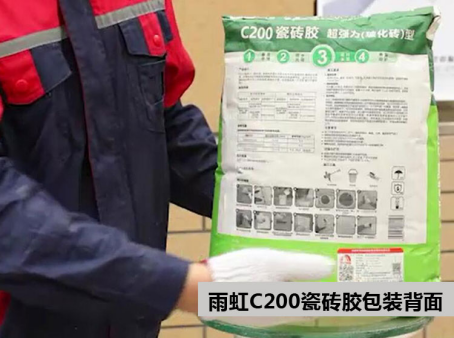雨虹C200瓷砖胶是以优质水泥为无机胶凝材料，精制砂为骨料，并添加多种功能型添加剂配制而成的粉状环保型瓷砖胶，适用于室内外铺贴吸水率大于0.2%的瓷砖，具有抗下滑、粘结强度高、不空鼓、施工方便等特点。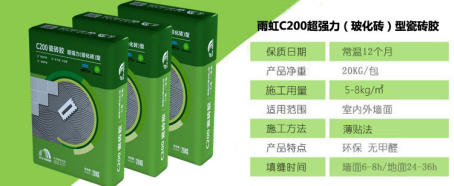雨虹C200瓷砖胶产品规格为20kg，外包装以绿色作为主色调，这也与这款产品具有良好的环境友好性相呼应，产品正面的2个认证LOGO非常明显：该产品通过了CTC中国建材检验认证、中国环境标志产品认证。在外包装的背面，是产品使用说明和出厂信息，方便在施工的时候为工长师傅提供参考。

雨虹C200瓷砖胶使用步骤

瓷砖铺贴施工环境温度应该选择在5℃-35℃之间，应避免在雨水、高温、大风、霜冻等天气施工。雨虹C200适用于水泥砂浆、混泥土、墙砖等稳定的基层，支持吸水率大于0.2%的瓷砖铺贴，并且根据使用场景不同，可以铺贴最大尺寸为600x600mm的瓷砖。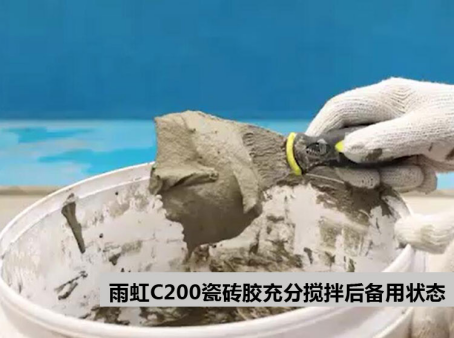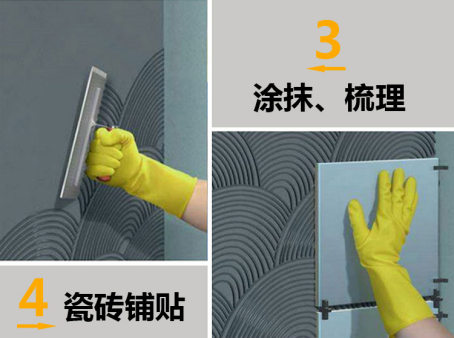使

使用雨虹C200瓷砖胶第一步是搅拌，在搅拌桶内按照合格证信息加水配比，用低速电动搅拌器搅拌至均匀无结块，放置3分钟后再次充分搅拌即可使用，施工使用最佳时间为充分搅拌完成后2个小时内。

雨虹C200瓷砖胶保水性测试

采用水泥砂浆贴砖时，因为水泥砂浆几乎没有保水性，导致砂浆将失水比较快，水泥水化不够充分，因此会影响粘结强度。另外部分瓷砖存在一定的吸水性，施工时很容易把水泥砂浆中的水分吸走，也会影响水泥水化，导致粘结强度降低。相对于水泥砂浆，雨虹C200瓷砖胶因为添加了多种功能型添加剂，因此具有良好的保水性，瓷砖胶中的水泥可以得到充分水化，因此可以获得更好的强度。

普通基层瓷砖铺贴测试对比

混凝土墙面是最常见的瓷砖铺贴基层，在测试中，我们选取了两种瓷砖胶进行对比铺贴。实验人员先在墙面上设置瓷砖铺贴基准线，然后使用某竞品瓷砖胶进行铺贴（右侧），随后用雨虹C200瓷砖胶在墙面（左侧）铺贴一块新瓷砖。铺贴完毕2分钟后，可以明显看到使用某竞品瓷砖胶铺贴的瓷砖已经下滑并偏离基准线，而且瓷砖呈现出不断下滑的趋势，相比较而言，使用雨虹C200瓷砖胶铺贴的瓷砖则相当稳固，由此可见，雨虹C200瓷砖胶在抗下滑性方面比竞品瓷砖胶要更加优秀。

防水基层瓷砖铺贴测试对比

已做防水的墙面，普遍存在于室内的卫生间/厨房，也常见于需要铺贴瓷砖的基层。与普通墙面施工步骤一致，用组合法将雨虹C200薄批于雨虹G101蓝色经典彩色超柔防水基层上，然后将两种不同类型瓷砖，釉面砖（左）、玻化砖（右）揉压于基层的瓷砖胶上于防水基层。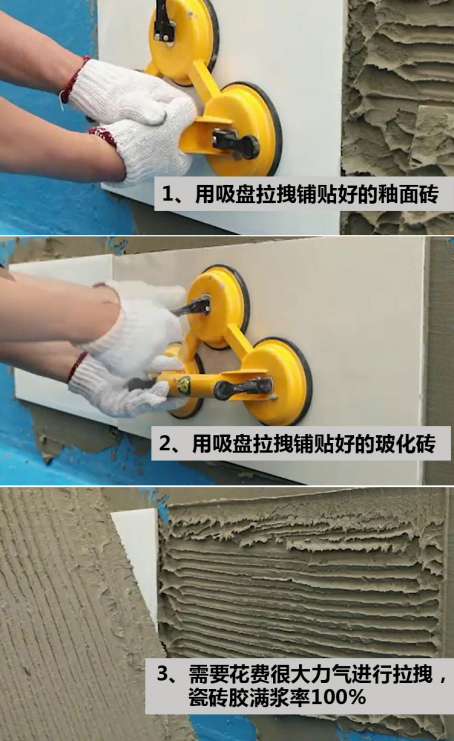完成铺贴以后，实验人员使用吸盘，分别尝试将已铺贴好的瓷砖拽下剥落，从实际过程中来看，用力拉拽吸盘器，可以看出，两种瓷砖拉拽下来都很费力。三爪吸盘器水平吸力约可达到1400N（牛顿），反映雨虹C200瓷砖胶的湿粘结强度较好，同时满浆率100%，与柔性防水也有很好的匹配性。

雨虹C200瓷砖胶拉拔道具体验

雨虹C200瓷砖胶铺贴的瓷砖，黏着力能达到什么程度？实验室制作了一套拉拔道具可供体验，侧面验证雨虹C200瓷砖胶的粘结强度。实验方法是从已粘贴好瓷砖的水泥砂浆小试块中，粘上拉拔头，引出重力绳以吊挂重物，此外，我们还邀请了2位“人高马大”的男性实验人员进行测试。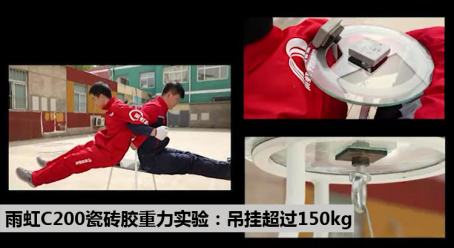从实际效果来看，雨虹C200瓷砖胶粘贴的瓷砖下可以轻松挂起总体重超过150kg的两位实验人员，实际受力面积仅为50mm*50mm。从这个实验中也可以明显看出，对于一般的家装铺贴瓷砖，雨虹C200完全能够胜任。

雨虹C200瓷砖胶粘接强度检测

前面我们定向了解了雨虹C200瓷砖胶铺贴瓷砖的湿粘接强度，也通过拉拔道具体验了雨虹C200瓷砖胶的优秀粘接力性能，但雨虹C200瓷砖胶铺贴的瓷砖粘接力能否定量测量出来呢？接下来我们将对两种试验条件下的雨虹瓷砖胶粘接强度进行测量。

第1种是室外已经粘贴超过150天的300mm*600mm的玻化砖墙面。实验人员首先对粘贴的瓷砖进行切割，取50mm*50mm瓷砖块用AB胶粘好金属拉拔头，采用高精度便携式拉拔仪对瓷砖进行拉拔强度测试，从拉拔仪的读数上看，试验数据为1456N，即0.58MPa，完全满足外墙饰面砖的粘结强度要求。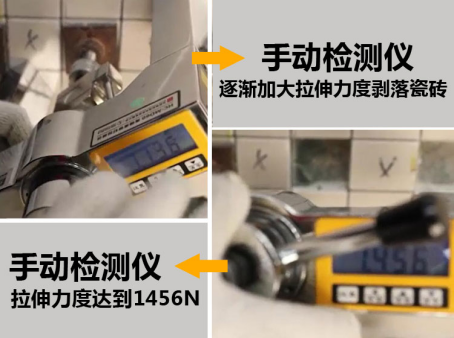第2种是室内标准条件下养护3天的雨虹C200瓷砖胶样块。从实验室拉拔仪器的显示数据可以看到，雨虹瓷砖胶3天原强度可达3112N，即1.2MPa，3天强度值就已远高于瓷砖胶C1标准要求（≥0.5）。评测总结：

造成瓷砖剥落的原因有多种，而选料不当又是其中的重要原因之一。从评测结果来看，雨虹C200瓷砖胶施工方便，比水泥砂浆拥有更好的锁水性能，因此在施工过程和长久使用中更加牢靠；雨虹C200在普通水泥基层和防水涂料基层上，都拥有较强的黏着力，不容易滑落和剥落。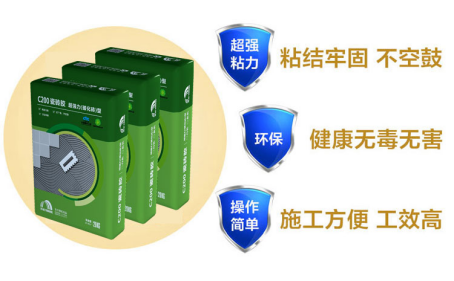此次评测也对雨虹C200瓷砖胶实际粘接强度进行了定性和定量测试，从结果来看，使用雨虹瓷砖胶粘贴的瓷砖，其拉拔强度均在0.5MPa以上，这相当于在贴好的600mm*600mm瓷砖下可以悬挂18吨的重物，两头大象也不怕。因此，使用雨虹C200瓷砖胶进行施工，再也不用担心会出现瓷砖剥落的情况了。

`声明：本文由入驻焦点开放平台的作者撰写，除焦点官方账号外，观点仅代表作者本人，不代表焦点立场错误信息举报电话： 400-099-0099，邮箱：jubao@vip.sohu.com，或点此进行意见反馈，或点此进行举报投诉。`A B C D E F G H J K L M N P Q R S T W X Y Z
A - B - C - D - E
• A
• 鞍山
• 安庆
• 安阳
• 安顺
• 安康
• 澳门
• B
• 北京
• 保定
• 包头
• 巴彦淖尔
• 本溪
• 蚌埠
• 亳州
• 滨州
• 北海
• 百色
• 巴中
• 毕节
• 保山
• 宝鸡
• 白银
• 巴州
• C
• 承德
• 沧州
• 长治
• 赤峰
• 朝阳
• 长春
• 常州
• 滁州
• 池州
• 长沙
• 常德
• 郴州
• 潮州
• 崇左
• 重庆
• 成都
• 楚雄
• 昌都
• 慈溪
• 常熟
• D
• 大同
• 大连
• 丹东
• 大庆
• 东营
• 德州
• 东莞
• 德阳
• 达州
• 大理
• 德宏
• 定西
• 儋州
• 东平
• E
• 鄂尔多斯
• 鄂州
• 恩施
F - G - H - I - J
• F
• 抚顺
• 阜新
• 阜阳
• 福州
• 抚州
• 佛山
• 防城港
• G
• 赣州
• 广州
• 桂林
• 贵港
• 广元
• 广安
• 贵阳
• 固原
• H
• 邯郸
• 衡水
• 呼和浩特
• 呼伦贝尔
• 葫芦岛
• 哈尔滨
• 黑河
• 淮安
• 杭州
• 湖州
• 合肥
• 淮南
• 淮北
• 黄山
• 菏泽
• 鹤壁
• 黄石
• 黄冈
• 衡阳
• 怀化
• 惠州
• 河源
• 贺州
• 河池
• 海口
• 红河
• 汉中
• 海东
• 怀来
• I
• J
• 晋中
• 锦州
• 吉林
• 鸡西
• 佳木斯
• 嘉兴
• 金华
• 景德镇
• 九江
• 吉安
• 济南
• 济宁
• 焦作
• 荆门
• 荆州
• 江门
• 揭阳
• 金昌
• 酒泉
• 嘉峪关
K - L - M - N - P
• K
• 开封
• 昆明
• 昆山
• L
• 廊坊
• 临汾
• 辽阳
• 连云港
• 丽水
• 六安
• 龙岩
• 莱芜
• 临沂
• 聊城
• 洛阳
• 漯河
• 娄底
• 柳州
• 来宾
• 泸州
• 乐山
• 六盘水
• 丽江
• 临沧
• 拉萨
• 林芝
• 兰州
• 陇南
• M
• 牡丹江
• 马鞍山
• 茂名
• 梅州
• 绵阳
• 眉山
• N
• 南京
• 南通
• 宁波
• 南平
• 宁德
• 南昌
• 南阳
• 南宁
• 内江
• 南充
• P
• 盘锦
• 莆田
• 平顶山
• 濮阳
• 攀枝花
• 普洱
• 平凉
Q - R - S - T - W
• Q
• 秦皇岛
• 齐齐哈尔
• 衢州
• 泉州
• 青岛
• 清远
• 钦州
• 黔南
• 曲靖
• 庆阳
• R
• 日照
• 日喀则
• S
• 石家庄
• 沈阳
• 双鸭山
• 绥化
• 上海
• 苏州
• 宿迁
• 绍兴
• 宿州
• 三明
• 上饶
• 三门峡
• 商丘
• 十堰
• 随州
• 邵阳
• 韶关
• 深圳
• 汕头
• 汕尾
• 三亚
• 三沙
• 遂宁
• 山南
• 商洛
• 石嘴山
• T
• 天津
• 唐山
• 太原
• 通辽
• 铁岭
• 泰州
• 台州
• 铜陵
• 泰安
• 铜仁
• 铜川
• 天水
• 天门
• W
• 乌海
• 乌兰察布
• 无锡
• 温州
• 芜湖
• 潍坊
• 威海
• 武汉
• 梧州
• 渭南
• 武威
• 吴忠
• 乌鲁木齐
X - Y - Z
• X
• 邢台
• 徐州
• 宣城
• 厦门
• 新乡
• 许昌
• 信阳
• 襄阳
• 孝感
• 咸宁
• 湘潭
• 湘西
• 西双版纳
• 西安
• 咸阳
• 西宁
• 仙桃
• 西昌
• Y
• 运城
• 营口
• 盐城
• 扬州
• 鹰潭
• 宜春
• 烟台
• 宜昌
• 岳阳
• 益阳
• 永州
• 阳江
• 云浮
• 玉林
• 宜宾
• 雅安
• 玉溪
• 延安
• 榆林
• 银川
• Z
• 张家口
• 镇江
• 舟山
• 漳州
• 淄博
• 枣庄
• 郑州
• 周口
• 驻马店
• 株洲
• 张家界
• 珠海
• 湛江
• 肇庆
• 中山
• 自贡
• 资阳
• 遵义
• 昭通
• 张掖
• 中卫

1室1厅1厨1卫1阳台

1
2
3
4
5

0
1
2

1

1

0
1
2
3报名成功，资料已提交审核A B C D E F G H J K L M N P Q R S T W X Y Z
A - B - C - D - E
• A
• 鞍山
• 安庆
• 安阳
• 安顺
• 安康
• 澳门
• B
• 北京
• 保定
• 包头
• 巴彦淖尔
• 本溪
• 蚌埠
• 亳州
• 滨州
• 北海
• 百色
• 巴中
• 毕节
• 保山
• 宝鸡
• 白银
• 巴州
• C
• 承德
• 沧州
• 长治
• 赤峰
• 朝阳
• 长春
• 常州
• 滁州
• 池州
• 长沙
• 常德
• 郴州
• 潮州
• 崇左
• 重庆
• 成都
• 楚雄
• 昌都
• 慈溪
• 常熟
• D
• 大同
• 大连
• 丹东
• 大庆
• 东营
• 德州
• 东莞
• 德阳
• 达州
• 大理
• 德宏
• 定西
• 儋州
• 东平
• E
• 鄂尔多斯
• 鄂州
• 恩施
F - G - H - I - J
• F
• 抚顺
• 阜新
• 阜阳
• 福州
• 抚州
• 佛山
• 防城港
• G
• 赣州
• 广州
• 桂林
• 贵港
• 广元
• 广安
• 贵阳
• 固原
• H
• 邯郸
• 衡水
• 呼和浩特
• 呼伦贝尔
• 葫芦岛
• 哈尔滨
• 黑河
• 淮安
• 杭州
• 湖州
• 合肥
• 淮南
• 淮北
• 黄山
• 菏泽
• 鹤壁
• 黄石
• 黄冈
• 衡阳
• 怀化
• 惠州
• 河源
• 贺州
• 河池
• 海口
• 红河
• 汉中
• 海东
• 怀来
• I
• J
• 晋中
• 锦州
• 吉林
• 鸡西
• 佳木斯
• 嘉兴
• 金华
• 景德镇
• 九江
• 吉安
• 济南
• 济宁
• 焦作
• 荆门
• 荆州
• 江门
• 揭阳
• 金昌
• 酒泉
• 嘉峪关
K - L - M - N - P
• K
• 开封
• 昆明
• 昆山
• L
• 廊坊
• 临汾
• 辽阳
• 连云港
• 丽水
• 六安
• 龙岩
• 莱芜
• 临沂
• 聊城
• 洛阳
• 漯河
• 娄底
• 柳州
• 来宾
• 泸州
• 乐山
• 六盘水
• 丽江
• 临沧
• 拉萨
• 林芝
• 兰州
• 陇南
• M
• 牡丹江
• 马鞍山
• 茂名
• 梅州
• 绵阳
• 眉山
• N
• 南京
• 南通
• 宁波
• 南平
• 宁德
• 南昌
• 南阳
• 南宁
• 内江
• 南充
• P
• 盘锦
• 莆田
• 平顶山
• 濮阳
• 攀枝花
• 普洱
• 平凉
Q - R - S - T - W
• Q
• 秦皇岛
• 齐齐哈尔
• 衢州
• 泉州
• 青岛
• 清远
• 钦州
• 黔南
• 曲靖
• 庆阳
• R
• 日照
• 日喀则
• S
• 石家庄
• 沈阳
• 双鸭山
• 绥化
• 上海
• 苏州
• 宿迁
• 绍兴
• 宿州
• 三明
• 上饶
• 三门峡
• 商丘
• 十堰
• 随州
• 邵阳
• 韶关
• 深圳
• 汕头
• 汕尾
• 三亚
• 三沙
• 遂宁
• 山南
• 商洛
• 石嘴山
• T
• 天津
• 唐山
• 太原
• 通辽
• 铁岭
• 泰州
• 台州
• 铜陵
• 泰安
• 铜仁
• 铜川
• 天水
• 天门
• W
• 乌海
• 乌兰察布
• 无锡
• 温州
• 芜湖
• 潍坊
• 威海
• 武汉
• 梧州
• 渭南
• 武威
• 吴忠
• 乌鲁木齐
X - Y - Z
• X
• 邢台
• 徐州
• 宣城
• 厦门
• 新乡
• 许昌
• 信阳
• 襄阳
• 孝感
• 咸宁
• 湘潭
• 湘西
• 西双版纳
• 西安
• 咸阳
• 西宁
• 仙桃
• 西昌
• Y
• 运城
• 营口
• 盐城
• 扬州
• 鹰潭
• 宜春
• 烟台
• 宜昌
• 岳阳
• 益阳
• 永州
• 阳江
• 云浮
• 玉林
• 宜宾
• 雅安
• 玉溪
• 延安
• 榆林
• 银川
• Z
• 张家口
• 镇江
• 舟山
• 漳州
• 淄博
• 枣庄
• 郑州
• 周口
• 驻马店
• 株洲
• 张家界
• 珠海
• 湛江
• 肇庆
• 中山
• 自贡
• 资阳
• 遵义
• 昭通
• 张掖
• 中卫• 手机• 分享
• 设计
免费设计
• 计算器
装修计算器
• 入驻
合作入驻
• 联系
联系我们
• 置顶
返回顶部